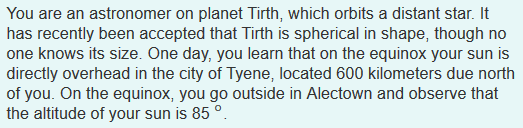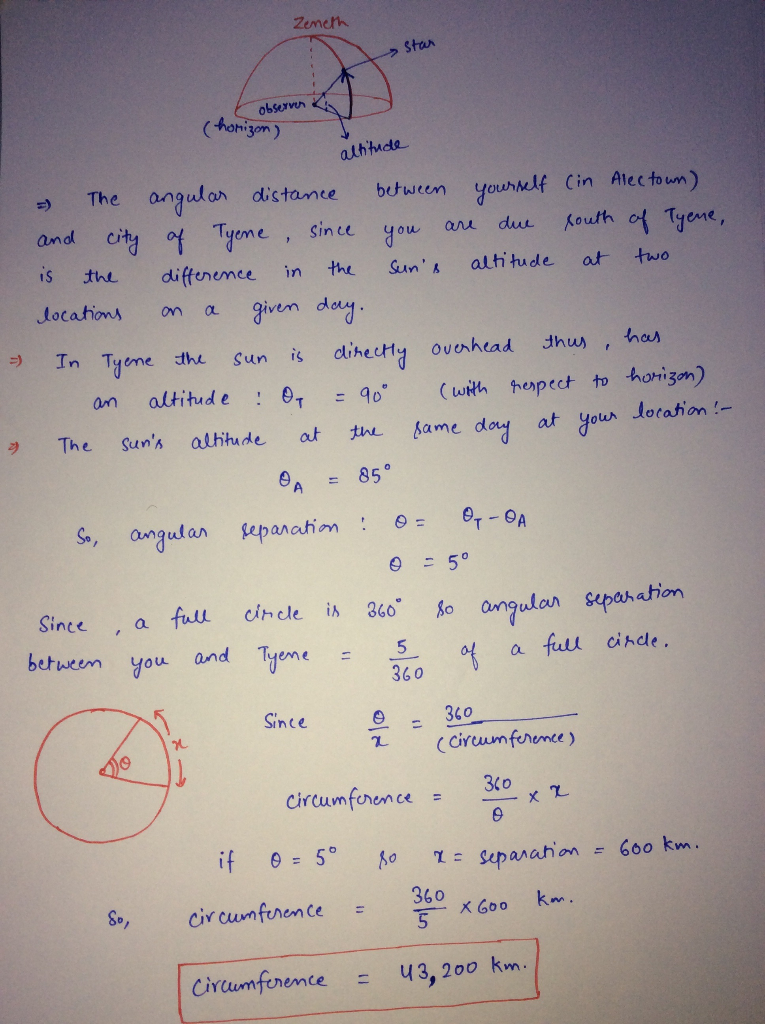# What is the circumference of tirth?What is the circumference of Tirth? You are an astronomer on planet Tirth, which orbits a distant star. It has recently been accepted that Tirth is spherical in shape, though no one knows its size. One day, you learn that on the equinox your sun is directly overhead in the city of Tyene, located 600 kilometers due north of you. On the equinox, you go outside in Alectown and observe that the altitude of your sun is 85°

Zeneth Star observer (horizon) altitude = The angular distance between yourself (in Alectown) and city of Tyene, since you are due south of Tyene, is the difference in the sun’s altitude at two locations on a given day. – In Tyene the sun is directly overhead thus, has I an altitude : Op = 90° ( with respect to horizon) & The sun’s altitude at the same day at your location! 0 = 85° So, angular reparation: 0= Op-OA 0 = 50 since , a full circle is 360° so angular separation between you and Tyene = 5 of a full circe. Since 9 = 360 (circumference) circumference – 360 x x if 0 = 50 so x = separation = 600km. circunference & 360 x 600 km. circumference = 43, 200 km. So,Also Read :   What does outstanding insurance transaction mean?